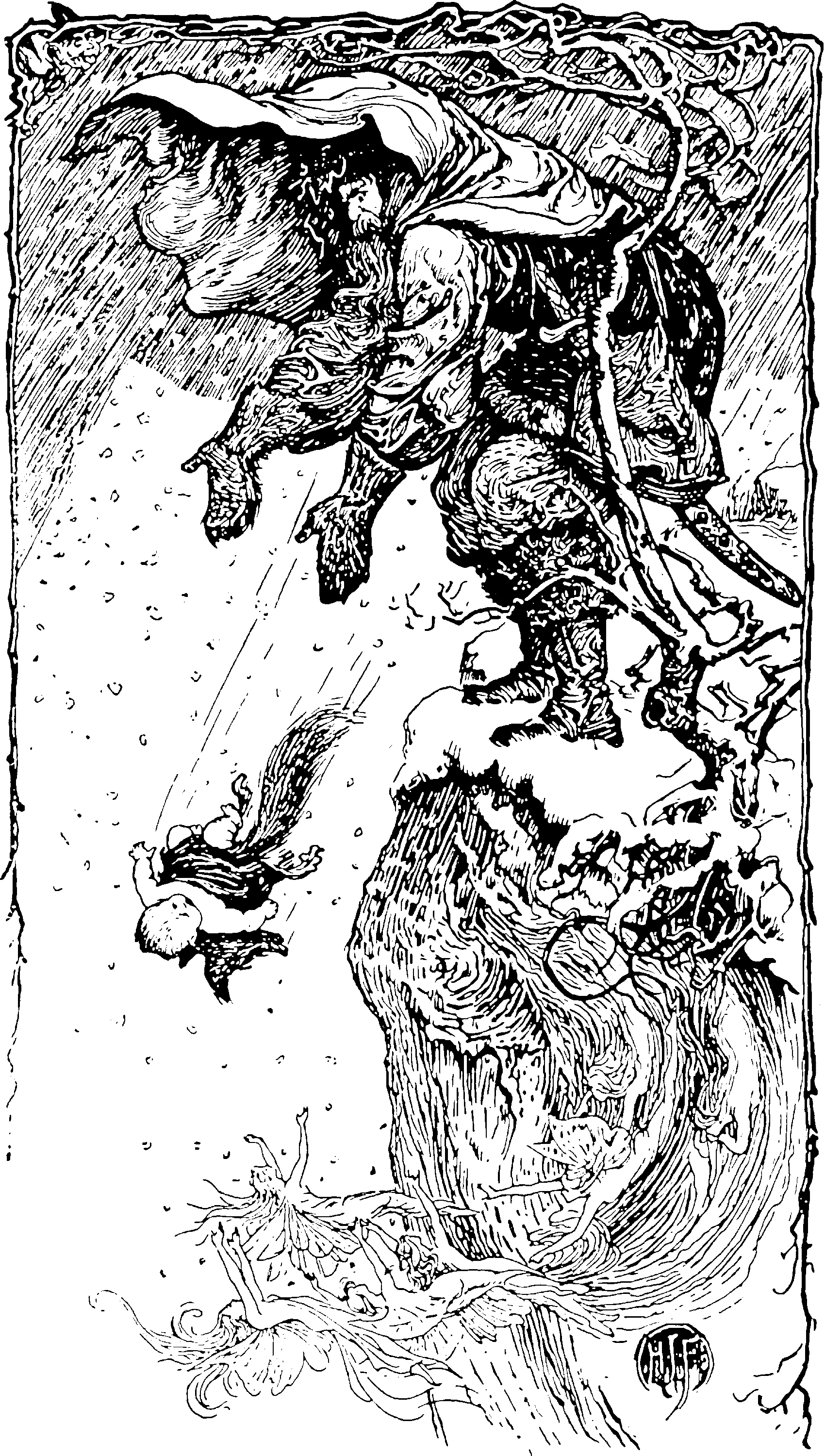# Quantitative risk measurement

## Mathematics of actuarial and financial disasterActuarial bread-and-butter. The mathematical study of measuring the chances of something terrible happening. This is usually a financial risk, but can also be extreme weather conditions, earthquakes, whatever. BTW, this is distinct from the “risk” in “statistical risk bounds”, which is the domain of statistical learning theory.

How do you evaluate how bad the worst cases are when deciding whether to do something? Generally this involved ignoring how good the best scenario is; Given financial history, probably that’s the niche to worry about filling. How do you trade off the badness and likeliness of the bad cases? This is about the risk measures themselves. For a useful class of distributions to use in this context it might be worth considering extreme value theory.

🏗 introduce risk coherence, and Discuss application to climate risk, and scheduling.

## Value-at-Risk

$$X$$ is a random variable the payoff of a portfolio at some future time, and our quantile of interest is $$0 < \alpha < 1$$.

The $$\alpha-\operatorname{VaR}$$ of an asset $$X$$ with cdf $$F$$ is, up to minus signs, an estimate of the icdf. It is defined as

\begin{aligned} \operatorname{VaR}_\alpha(X) &:= \inf\{x\in\mathbb{R}:\mathbb{P}(X<-x)\leq 1-\alpha\}\\ &= \inf\{x\in\mathbb{R}:1-F(-x)\geq \alpha\}\\ \end{aligned}

## Expected shortfall

“the expected loss of portfolio value given that a loss is occurring at or above the $$q$$-quantile.”

The expected shortfall (ES) is defined

$ES_{\alpha} := \frac{1}{\alpha}\int_0^{\alpha} \operatorname{VaR}_{\gamma}(X)d\gamma\\ = -\frac{1}{\alpha}\left(E[X \ 1_{\{X \leq x_{\alpha}\}}] + x_{\alpha}(\alpha - \mathbb{P}[X \leq x_{\alpha}])\right)$

where VaR is Value At Risk, and where $$x_{\alpha} = \inf\{x \in \mathbb{R}: P(X \leq x) \geq \alpha\}$$

It is also known as the Conditional Value-at-Risk, $$\alpha-\operatorname{CVaR}.$$

According to Wikipedia, I might care about the dual representation, $$ES_{\alpha} = \inf_{Q \in \mathcal{Q}_{\alpha}} E^Q[X]$$ with $$\mathcal{Q}_{\alpha}$$ the set of probability measures absolutely continuous with respect to the physical measure $$P$$, such that $$\frac{dQ}{dP} \leq \alpha^{-1}$$ almost surely.

…Why might I care about that again?

TBC## G-expectation

I don’t understand this yet, but Shige Peng just gave a talk wherein he argued that the generalised, sublinear expectation operator derived from distributional uncertainty, generate coherent risk measures, although it is not immediately obvious to me how this works. See, e.g. Shige Peng (2004).

## Sensitivity to parameters of risk measures

SWIM is a method for analysis of sensitivity for parameter assumptions in risk measures. (R package) I might use this article as a point of entry to this field if I need it.

An efficient sensitivity analysis for stochastic models based on Monte Carlo samples. Provides weights on simulated scenarios from a stochastic model, such that stressed random variables fulfil given probabilistic constraints (e.g. specified values for risk measures), under the new scenario weights. Scenario weights are selected by constrained minimisation of the relative entropy to the baseline model.

## Rosenblatt transform

Mentioned mnemonically because it seems to arise in QRM all the time. The Rosenblatt transform is the one that takes a vector random variate with a known (continuous) joint distribution and transforms it to a uniform distribution over the unit hypercube.

## Knightian risk

See black swans.

### No comments yet. Why not leave one?

GitHub-flavored Markdown & a sane subset of HTML is supported.